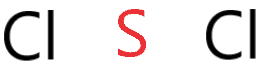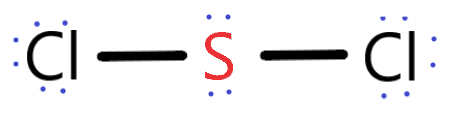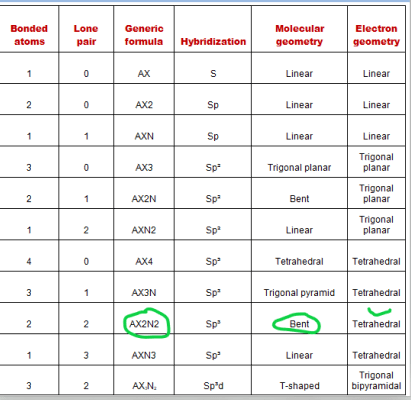# Sulfur dichloride (SCl2) Lewis dot Structure, Molecular Geometry, Polarity, Bond angle, hybridization

Home  > Chemistry Article > Scl2 lewis structure and its molecular geometrySulfur dichloride is a chemical compound with a pungent smell and appearance like Red liquid. Sulfur dichloride is mostly used in organic synthesis and its formula is SCl2.

So, in this article, we will learn about the Sulfur dichloride (SCl2) lewis dot structure, its molecular geometry, polar or non-polar, and hybridization.

Some other properties of Sodium dichloride

• The boiling point of SCl2 is 59°C.
• The melting point of SCl2 is -121°C.
• Density is 1.621 g.cm-3
• The Refractive index is 1.5570
• Molar mass is 102.97 g·mol−1
 Name of Molecule Sodium dichloride Chemical formula SCl2 Molecular geometry of SCl2 Bent Electron geometry of SCl2 Tetrahedral Hybridization Sp³ Bond angle 103º Total Valence electron for SCl2 20 The formal charge of SCl2 0

SCl2 can be produced by two processes by using sulfur or disulfur dichloride.

The first process is by reacting sulfur with dichloride then it formed disulfur dichloride.

⇒ S8 + 4Cl2 → 4 S2Cl2

And the Second process includes reacting disulfur dichloride with dichloride and it will form Sulfur dichloride.

⇒ S2Cl2 + Cl2 → 2 SCl2

Page Contents

## How to draw Lewis Structure of SCl2?

SCl2 lewis structure contains one sulfur and two chlorine atom. Sulfur being the less electronegative atom than chlorine atom is placed at the center in lewis’s diagram and chlorine is spaced evenly around it.

There is two lone pair present on the central atom and this central atom attached to two bonded pair in SCl2 lewis structure.

## Steps to draw electron dot structure or lewis structure of SCl2

Step 1: First you should count the total number of valence electrons in SCl2. For knowing valence electron you should know the group number of its compound.

So, by observing the periodic table we know sulfur belongs to the 16th group and chlorine belongs to the 17th group.

⇒ Sulfur Valence electron = 6

⇒ Chlorine valence electron = 7

∴ Total number of valence electron available for SCl2 lewis structure = 6 + 7(2) = 20 valence electrons.Step 2: Now find the least electronegative element then placed it at the center in lewis’s diagram and other atoms spaced evenly around it.

So, we know sulfur has the least electronegativity in the SCl2 compound because the electronegativity increase from left to right in the periodic table.

Therefore, place sulfur in the central position, and chlorine spread around it.Step 3: In the third step, draw a single bond to connect the outer atom(chlorine) to the central atom(sulfur) for further drawing the SCl2 lewis structure.As in the shown figure, we draw the single bond from the left and right side chlorine atoms to the central atom. Now count the valence used in the above structure.

A single bond contains two electrons, and, in the above structure, two single bonds are used, hence, 4 valence electrons are used in the above structure from the total of 20 valence electrons that is available for drawing the lewis structure of SCl2.

∴ (20 – 4) = 16 valence electrons

So, we are left with 16 valence electrons more.

Step 4: Now complete the octet with the remaining electron for the surrounding atoms first after that if any electron is left then placed it around the central atom.

As octet means having 8 electrons in valency share. So, each atom needs 8 electrons in the outer shell to satisfy the rule of the octet.

So, place the remaining valence electron on the outer atom(chlorine) first to complete its octet.As shown in the figure, we place 6 electrons around the chlorine atoms on both sides as they already sharing 2 electrons with a single bond, hence, chlorine atoms completed their octet.

Step 5: This is the final step to draw the lewis dot structure for SCl2.

As in the fourth step structure, we use 16 valence electrons (including 4 single bond electrons + 6 electrons on each chlorine atom) so, we are left with only 4 electrons which we have to place around the central atom so that sulfur also completes its octet rule.### SCl2 Lewis dot structure

So, this is the simple procedure to draw the lewis structure or electron dot structure for Sulfur dichloride (SCl2)

## What are the Molecular Geometry or Shape of SCl2?

Molecular geometry is the way of arrangement of atoms in molecules. By looking at the lewis structure of SCl2 many assume that it has a linear shape but they are wrong Because it is one of the biggest limitations in Lewis’s theory as it does not help to determine the accurate shape of molecules.

So, for overcoming this problem VSEPR (Valence shell electron pair repulsion) theory comes to determine the molecular shape of chemical compounds.

The molecular geometry of SCl2 is bent because it contains two lone pairs on the central atom that tries to repel each other and bonded pairs of electrons around it, as a result, it pushes down the bonded atoms giving the bent geometry structure.

Also, there are asymmetric charges distribute around the central atom due to this. The repulsion generated by these lone pair electrons on the central atom causes the SCl2 molecule to become bent.### SCL2 molecular geometry or shape

The bond angle of SCl2 is approx 103º.

The electron pair geometry of SCl2 is tetrahedral as it considers lone pair on the central atom as well as bonded pair around it.

The generic formula for SCl2 is AX2N2 according to the VSEPR theory and it’s the chart.SCl2 molecular geometry is very similar to H2O but its bond angle is slightly lower than H2O because of the lone pair. As higher the lone pair, the smaller is the bond angle and the bond length of SCl2 is also higher than H2O.

## What is the Hybridization of SCl2?

To calculate the hybridization of SCl2 follows some simple steps.

Step 1: Determine the number of atoms attached to the central atom as we know the central atom is that atom that has the least electronegativity.

So, Sulfur (S) has the least electronegativity therefore by looking at SCl2 we determined that only 2 atoms are attached to the central atom.

The number of attached atoms to sulfur is = 2

Step 2: Now find the lone pair of central atoms (sulfur)

As the Valence electron for sulfur is 6 and from 6 electrons it shares only 2 electrons to make bonding with chlorine atoms. So, we left with 4 electrons which make 2 lone pairs.

Step 3: This is the final step to determine the hybridization of SCl2.

Hybridization = Number of atoms attached + Number of lone pairs

= 2 + 2

= 4 which makes the hybridization of SCl2 is sp3.

## Sulfur dichloride Polarity: is SCl2 polar or non-polar?

If you have basic knowledge of polarity, dipole moment, and electronegativity then you can already know the answer of Is SCl2 polar or non-polar?

SCl2 is polar because of its asymmetrical shape and non-uniform charge distribution around the atoms.

To understand the depth of polar nature of SCl2 we have to take an overview of electronegativity, dipole moment, and geometrical shape.

1.Electronegativity

This is the big factor in knowing the polarity of SCl2 as the difference between the electronegativity of atoms is directly proportional to the polarity of molecules.

Electronegativity of chlorine = 3.16

Electronegativity of sulfur = 2.58

And the difference of these atoms makes electronegativity greater than 0.5 which directly makes SCl2 polar in nature.

2. Dipole moment

This factor can help us to know the strength of polarity. As greater the dipole moment more is the polarity of the molecule.

As SCl2 shape is asymmetric in nature. Because of this dipole moment of SCl2 does not cancel each other.

⇒ Dipole moment formula =  charge on the atoms * the distance between them

∴ D = Q × R

Net dipole moment of SCl2 is = 0.54d

3. Geometrical structure

The shape is also a big factor to determine whether SCl2 is polar or non-polar

More the asymmetrical shape of molecules greater is the dipole moment as symmetrical structure dipole moment can easily be canceled out but asymmetrical or bent structure provides some dipole moment which makes to molecules polar in nature.

## Uses of sulfur dichloride

1. Sulfur dichloride is an oily liquid with a strong irritating odor used in the manufacture of organic chemicals.
2. It is used in insecticides, and for manufacturing synthetic rubber.
3. It is used as a chlorinating agent in various industries.
4. It is used for hardening softwoods.
5. It is used to make vulcanized oils and rubber.
6. It also uses to purify sugar juices.

## Summary

So, we learned in this article about an important thing like SCl2 lewis structure, molecular geometry, hybridization, its polarity in detail, and in the very simple and friendly language which will help you in long run.

Let’s take a quick overview of SCl2

SCl2 molecular geometry = Bent or angular

SCl2 bond angle = 103º

Dipole moment of SCl2 = 0.54d

Hybridization of sulfur dichloride = sp3

## FAQ

### What is the oxidation number of SCl2?

The Sulfur(S) oxidation number is = +2

The chlorines oxidation number is = -1

And SCl2 oxidation number is = 0

### What is the electron configuration for Sulfur and Chlorine?

As we know sulfur contain 16 electrons around the atom and Chlorine contain 17 electrons so put these electrons in orbitals according to the sequence

Hence sulfur electron configuration is 1s22s22p63s23p

And chlorine electron configuration is 1s22s22p63s23p5

### Is sulfur dichloride is ionic or covalent?

As SCl2 is a polar molecule and electronegativity is great affects the compound for knowing its ionic or covalent bond.

If the difference of electronegativity of atoms in the Pauling scale is more than 1.9 then the bond is ionic.

But for SCl2 its electronegativity is less than 1.9 hence sulfur dichloride is covalent.

Sulfur Electronegativity = 2.58

Chlorine Electronegativity = 3.16

And the difference of electronegativity of Sulfur and Chlorine in SCl2 is = 0.58

### Why SCl2 is polar and has a bent geometrical structure?

SCl2 is polar because of its asymmetrical shape and SCl2 has a bent molecular structure because of the lone pair present on the sulfur central atom which causes non-uniform distribution and makes its structure bent or V-shaped.

Net dipole moment and electronegativity are also responsible for the SCl2 polarity nature.

### What are the electron and molecular geometry of SCl2?

The electron geometry of SCl2 is determined by the steric number which is equal to the number of sigma bonds present around the atom + the number of lone pairs on the atoms. Sulfur is the central atom in the Lewis structure of SCl2 that has a steric number equal to 4, hence the electron geometry of SCl2 is tetrahedral.

The molecular geometry of SCl2 is simply determined by considering only the number of bonded atoms, and as per VSEPR theory, its shape is bent.

##### Subscribe to Blog via Email

Join 2 other subscribers

Share it...